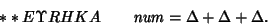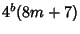## Fermat's Polygonal Number Theorem

In 1638, Fermatproposed that every Positive Integer is a sum of at most three Triangular Numbers, four Square Numbers, five Pentagonal Numbers, and-Polygonal Numbers. Fermat claimed to have a proof of this result, although Fermat's proof has never been found. Gaußproved the triangular case, and noted the event in his diary on July 10, 1796, with the notationThis case is equivalent to the statement that every number of the formis a sum of three Odd Squares (Duke 1997). More specifically, a number is a sum of three Squares Iff it is not of the formfor, as first proved by Legendrein 1798.

Eulerwas unable to prove the square case of Fermat's theorem, but he left partial results which were subsequently used by Lagrange. The square case was finally proved by Jacobiand independently by Lagrangein 1772. It is therefore sometimes known as Lagrange's Four-Square Theorem. In 1813, Cauchyproved the proposition in its entirety.

References

Cassels, J. W. S. Rational Quadratic Forms. New York: Academic Press, 1978.

Conway, J. H.; Guy, R. K.; Schneeberger, W. A.; and Sloane, N. J. A. The Primary Pretenders.'' Acta Arith. 78, 307-313, 1997.

Duke, W. Some Old Problems and New Results about Quadratic Forms.'' Not. Amer. Math. Soc. 44, 190-196, 1997.

Shanks, D. Solved and Unsolved Problems in Number Theory, 4th ed. New York: Chelsea, pp. 143-144, 1993.

Smith, D. E. A Source Book in Mathematics. New York: Dover, p. 91, 1984.